Aa Bb Cc Dd Ee Ff Gg Hh Ii Jj Kk Ll Mm Nn Oo Pp Qq Rr Ss Tt Uu Vv Ww Xx Yy Zz

Bb

binary system

• a number system using the base number 2.
• most computers and other electronic devices use the binary system.
• binary numbers are written using the digits 0 and 1 to express each number.
• binary digits are called 'bits'.

EXAMPLE: 1100 = 12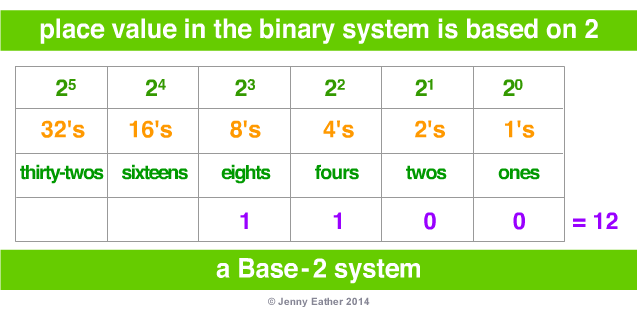base numbers and number systems

• a base number is the basis of a place value number system,
• successive powers of the base number
are used for each column.
• the decimal system uses 10 as its base number
so it is called a Base-10 system.
• the binary system uses 2 as its base number so
it is called a Base-2 system.

EXAMPLE: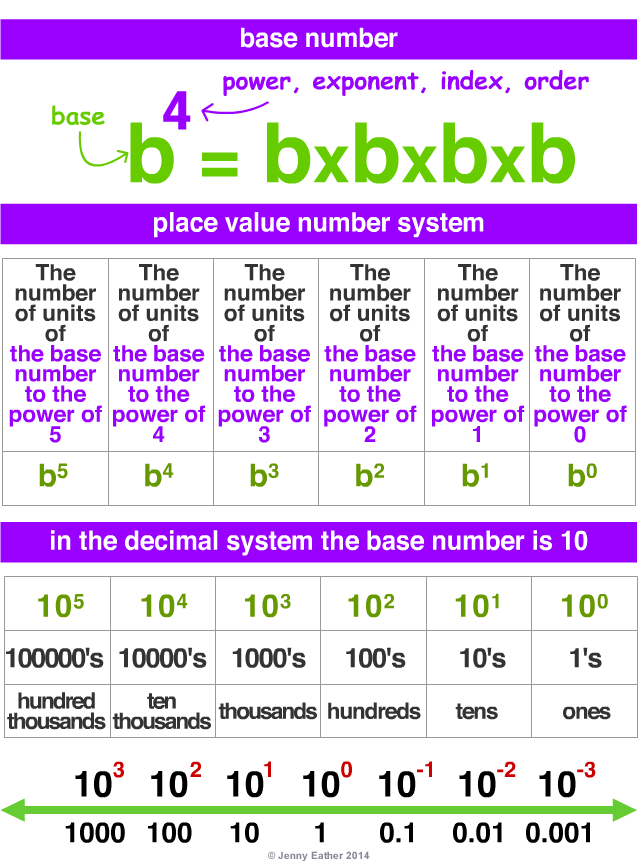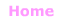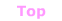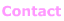© Jenny Eather 2014. All rights reserved.